### 四、一阶非线性方程

[完全解・通解・奇异解一阶非线性方程的一般形式为若一阶偏微分方程的解包含任意n个独立的常数，则称这样的解为完全解(全积分).

V ( x1, x2xn , u , c1 , c2cn ) = 0为方程的完全解，从以两个独立变量为例说明完全解与通解、奇异解的关系，设方程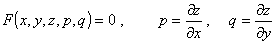V (x,y,z,a,b)=0          ( a,b为任意常数),利用常数变易法把a,b看作x, y的函数，将V (x,y,z,a,b)=0求关于x, y的偏导数，得V=0联立可确定a,b.有三种情况：

1°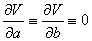，将其与V(x,y,z,a,b)=0联立可确定不含任意常数的奇异解.

2°，即回到完全解.

3°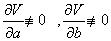时，必有，这时，如果不属于情形2° ，则ab存在函数关系：b=(a)，这里为任意可微函数，并从方程V(x,y,z,a,b)=0消去a,b可确定方程的通解.

定理  偏微分方程的任何解包含在完全解内或通解内或奇异解内.

[特征方程・特征带・特征曲线・初积分在一阶非线性方程：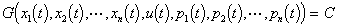[求完全解的拉格朗日－恰比方法考虑两个变量的情况.

对于方程F(x,y,z,p,q)=0，选择使雅可比式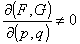的一个初积分G(x,y,z,p,q).解方程组(a为任意常数)

p(x,y,z,a)q(x,y,z,a).则方程

dz=pdx+qdy

求方程的完全解.

方程的特征方程为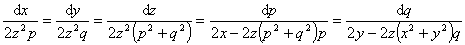解方程组(a为任意常数)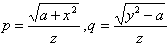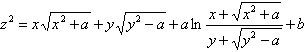(b为任意常数)

[某些容易求完全解的方程]

1°  仅含p,q的方程F(p,q)=0

G=p是特征方程的一个初积分.F(p,q)=0p=a(a为任意常数)q=(a)，积分

z=ax+(a)y+b    (b为任意常数)

2°  不显含x,y的方程F(z,p,q)=0

特征方程为dz=(z,a)dx+a(z,a)dy(b为任意常数)

3°  变量分离形式的方程pi=i(xi,ai)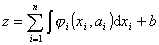[克莱罗方程方程ci微分得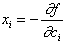(i=1,2,,n)

求方程zxpyqpq=0的完全解和奇异解.

这是克莱罗方程，它的完全解是

z=ax+by+ab

a,b微分，得x=b,y=a消去a,b得奇异解

z=xy

[发甫方程方程

`P(x,y,z)dx+Q(x,y,z)dy+R(x,y,z)dz=0                                   (1)`

1°  方程完全可积的充分必要条件   当且仅当P,Q,R满足条件

`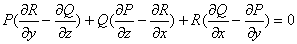(2)`

dU1=(Pdx+Qdy+Rdz)

U1(x,y,z)=c

特别，当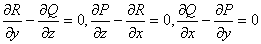时，存在一个函数U(x,y,z)满足所以方程的通解为

U(x,y,z)=c

所以完全可积的发甫方程的通解是一单参数的曲面族.

定理  设对于发甫方程(1)在某区域D上的完全可积条件(2)成立，则对D内任一点M(x,y,z)一定有方程的积分曲面通过，而且只有一个这样的积分曲面通过.

2°  方程积分曲面的求法

设完全可积条件(2)成立.为了构造积分曲面，把z看成x,y的函数(R(x,y,z)0)，于是原方程化为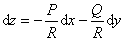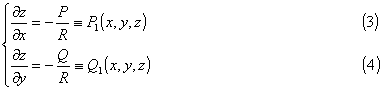把方程(3)中的y看成参变量，积分后得一个含有常数的通解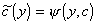c为任意常数，代回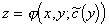中即得发甫方程的积分曲面

z=(x,y,(y,c))

由于发甫方程关于x,y,z的对称性，在上面的讨论中，也可把xy看成未知函数，得到同样的结果.

求方程yzdx+2xzdy+xydz=0的积分曲面族.

容易验证完全可积条件成立，显然存在一个积分因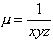用它乘原方程得xy2z=c

也可把方程化为等价的方程组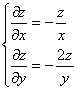y看成参变量积分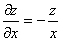得通解xy2z=c